Anzeige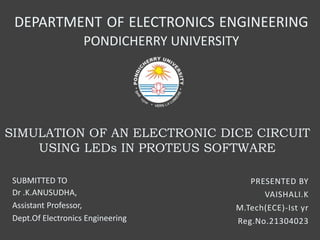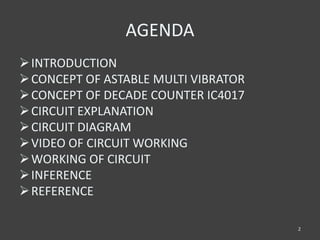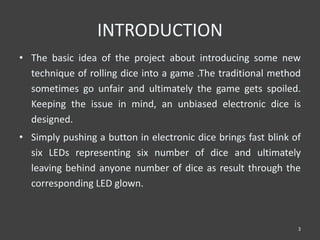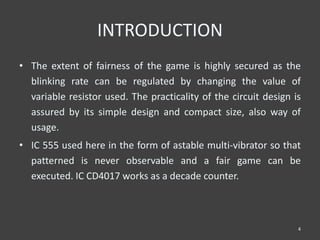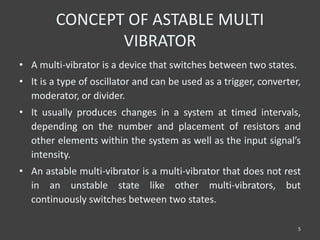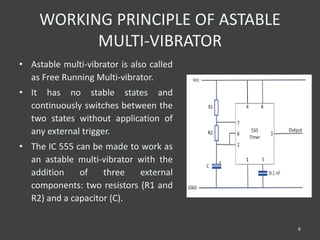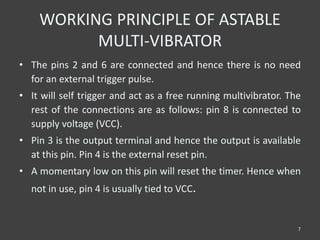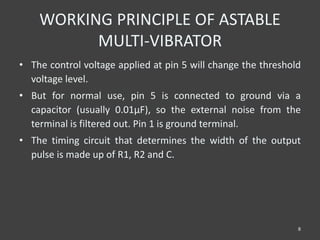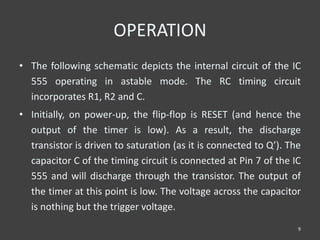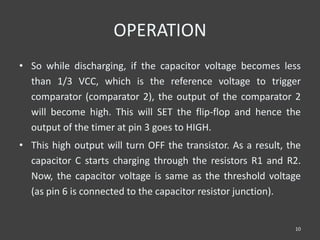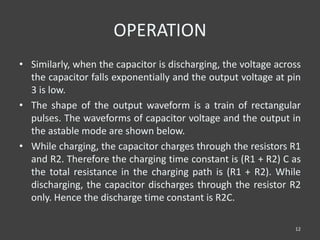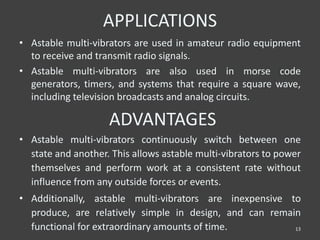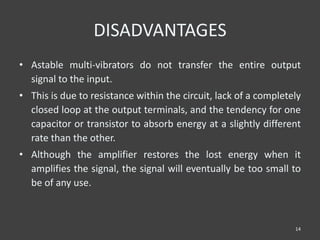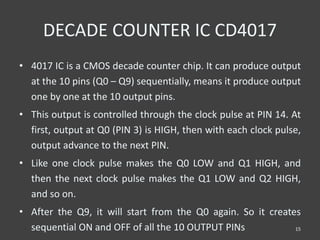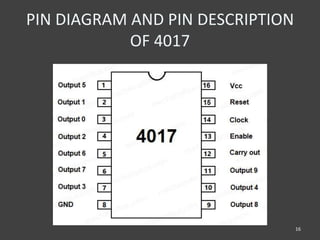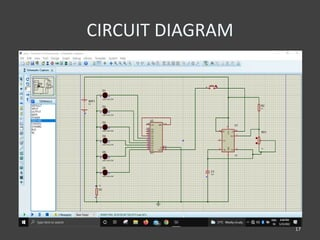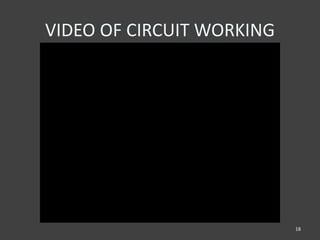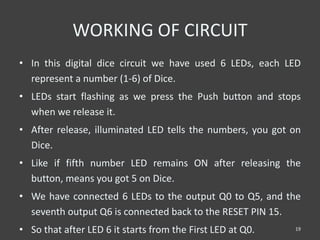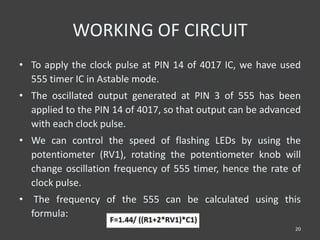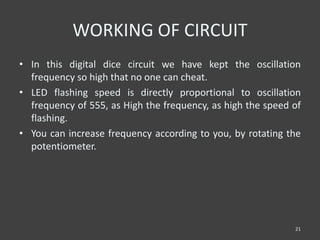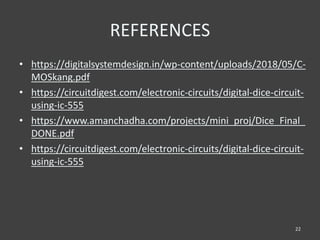1 von 23
Anzeige

### SIMULATION OF AN ELECTRONIC DICE CIRCUIT USING LEDs IN PROTEUS SOFTWARE

1. SIMULATION OF AN ELECTRONIC DICE CIRCUIT USING LEDs IN PROTEUS SOFTWARE PRESENTED BY VAISHALI.K M.Tech(ECE)-Ist yr Reg.No.21304023 DEPARTMENT OF ELECTRONICS ENGINEERING PONDICHERRY UNIVERSITY SUBMITTED TO Dr .K.ANUSUDHA, Assistant Professor, Dept.Of Electronics Engineering
2. AGENDA INTRODUCTION CONCEPT OF ASTABLE MULTI VIBRATOR CONCEPT OF DECADE COUNTER IC4017 CIRCUIT EXPLANATION CIRCUIT DIAGRAM VIDEO OF CIRCUIT WORKING WORKING OF CIRCUIT INFERENCE REFERENCE 2
3. INTRODUCTION • The basic idea of the project about introducing some new technique of rolling dice into a game .The traditional method sometimes go unfair and ultimately the game gets spoiled. Keeping the issue in mind, an unbiased electronic dice is designed. • Simply pushing a button in electronic dice brings fast blink of six LEDs representing six number of dice and ultimately leaving behind anyone number of dice as result through the corresponding LED glown. 3
4. INTRODUCTION • The extent of fairness of the game is highly secured as the blinking rate can be regulated by changing the value of variable resistor used. The practicality of the circuit design is assured by its simple design and compact size, also way of usage. • IC 555 used here in the form of astable multi-vibrator so that patterned is never observable and a fair game can be executed. IC CD4017 works as a decade counter. 4
5. CONCEPT OF ASTABLE MULTI VIBRATOR • A multi-vibrator is a device that switches between two states. • It is a type of oscillator and can be used as a trigger, converter, moderator, or divider. • It usually produces changes in a system at timed intervals, depending on the number and placement of resistors and other elements within the system as well as the input signal’s intensity. • An astable multi-vibrator is a multi-vibrator that does not rest in an unstable state like other multi-vibrators, but continuously switches between two states. 5
6. WORKING PRINCIPLE OF ASTABLE MULTI-VIBRATOR • Astable multi-vibrator is also called as Free Running Multi-vibrator. • It has no stable states and continuously switches between the two states without application of any external trigger. • The IC 555 can be made to work as an astable multi-vibrator with the addition of three external components: two resistors (R1 and R2) and a capacitor (C). 6
7. WORKING PRINCIPLE OF ASTABLE MULTI-VIBRATOR • The pins 2 and 6 are connected and hence there is no need for an external trigger pulse. • It will self trigger and act as a free running multivibrator. The rest of the connections are as follows: pin 8 is connected to supply voltage (VCC). • Pin 3 is the output terminal and hence the output is available at this pin. Pin 4 is the external reset pin. • A momentary low on this pin will reset the timer. Hence when not in use, pin 4 is usually tied to VCC. 7
8. WORKING PRINCIPLE OF ASTABLE MULTI-VIBRATOR • The control voltage applied at pin 5 will change the threshold voltage level. • But for normal use, pin 5 is connected to ground via a capacitor (usually 0.01µF), so the external noise from the terminal is filtered out. Pin 1 is ground terminal. • The timing circuit that determines the width of the output pulse is made up of R1, R2 and C. 8
9. OPERATION • The following schematic depicts the internal circuit of the IC 555 operating in astable mode. The RC timing circuit incorporates R1, R2 and C. • Initially, on power-up, the flip-flop is RESET (and hence the output of the timer is low). As a result, the discharge transistor is driven to saturation (as it is connected to Q’). The capacitor C of the timing circuit is connected at Pin 7 of the IC 555 and will discharge through the transistor. The output of the timer at this point is low. The voltage across the capacitor is nothing but the trigger voltage. 9
10. OPERATION • So while discharging, if the capacitor voltage becomes less than 1/3 VCC, which is the reference voltage to trigger comparator (comparator 2), the output of the comparator 2 will become high. This will SET the flip-flop and hence the output of the timer at pin 3 goes to HIGH. • This high output will turn OFF the transistor. As a result, the capacitor C starts charging through the resistors R1 and R2. Now, the capacitor voltage is same as the threshold voltage (as pin 6 is connected to the capacitor resistor junction). 10
11. OPERATION • While charging, the capacitor voltage increases exponentially towards VCC and the moment it crosses 2/3 VCC, which is the reference voltage to threshold comparator (comparator 1), its output becomes high. • As a result, the flip-flop is RESET. The output of the timer falls to LOW. This low output will once again turn on the transistor which provides a discharge path to the capacitor. Hence the capacitor C will discharge through the resistor R2. And hence the cycle continues. • Thus, when the capacitor is charging, the voltage across the capacitor rises exponentially and the output voltage at pin 3 is high. 11
12. OPERATION • Similarly, when the capacitor is discharging, the voltage across the capacitor falls exponentially and the output voltage at pin 3 is low. • The shape of the output waveform is a train of rectangular pulses. The waveforms of capacitor voltage and the output in the astable mode are shown below. • While charging, the capacitor charges through the resistors R1 and R2. Therefore the charging time constant is (R1 + R2) C as the total resistance in the charging path is (R1 + R2). While discharging, the capacitor discharges through the resistor R2 only. Hence the discharge time constant is R2C. 12
13. APPLICATIONS • Astable multi-vibrators are used in amateur radio equipment to receive and transmit radio signals. • Astable multi-vibrators are also used in morse code generators, timers, and systems that require a square wave, including television broadcasts and analog circuits. 13 ADVANTAGES • Astable multi-vibrators continuously switch between one state and another. This allows astable multi-vibrators to power themselves and perform work at a consistent rate without influence from any outside forces or events. • Additionally, astable multi-vibrators are inexpensive to produce, are relatively simple in design, and can remain functional for extraordinary amounts of time.
14. DISADVANTAGES • Astable multi-vibrators do not transfer the entire output signal to the input. • This is due to resistance within the circuit, lack of a completely closed loop at the output terminals, and the tendency for one capacitor or transistor to absorb energy at a slightly different rate than the other. • Although the amplifier restores the lost energy when it amplifies the signal, the signal will eventually be too small to be of any use. 14
15. DECADE COUNTER IC CD4017 • 4017 IC is a CMOS decade counter chip. It can produce output at the 10 pins (Q0 – Q9) sequentially, means it produce output one by one at the 10 output pins. • This output is controlled through the clock pulse at PIN 14. At first, output at Q0 (PIN 3) is HIGH, then with each clock pulse, output advance to the next PIN. • Like one clock pulse makes the Q0 LOW and Q1 HIGH, and then the next clock pulse makes the Q1 LOW and Q2 HIGH, and so on. • After the Q9, it will start from the Q0 again. So it creates sequential ON and OFF of all the 10 OUTPUT PINs 15
16. PIN DIAGRAM AND PIN DESCRIPTION OF 4017 16
17. CIRCUIT DIAGRAM 17
18. VIDEO OF CIRCUIT WORKING 18
19. WORKING OF CIRCUIT • In this digital dice circuit we have used 6 LEDs, each LED represent a number (1-6) of Dice. • LEDs start flashing as we press the Push button and stops when we release it. • After release, illuminated LED tells the numbers, you got on Dice. • Like if fifth number LED remains ON after releasing the button, means you got 5 on Dice. • We have connected 6 LEDs to the output Q0 to Q5, and the seventh output Q6 is connected back to the RESET PIN 15. • So that after LED 6 it starts from the First LED at Q0. 19
20. WORKING OF CIRCUIT • To apply the clock pulse at PIN 14 of 4017 IC, we have used 555 timer IC in Astable mode. • The oscillated output generated at PIN 3 of 555 has been applied to the PIN 14 of 4017, so that output can be advanced with each clock pulse. • We can control the speed of flashing LEDs by using the potentiometer (RV1), rotating the potentiometer knob will change oscillation frequency of 555 timer, hence the rate of clock pulse. • The frequency of the 555 can be calculated using this formula: 20
21. WORKING OF CIRCUIT • In this digital dice circuit we have kept the oscillation frequency so high that no one can cheat. • LED flashing speed is directly proportional to oscillation frequency of 555, as High the frequency, as high the speed of flashing. • You can increase frequency according to you, by rotating the potentiometer. 21
22. REFERENCES • https://digitalsystemdesign.in/wp-content/uploads/2018/05/C- MOSkang.pdf • https://circuitdigest.com/electronic-circuits/digital-dice-circuit- using-ic-555 • https://www.amanchadha.com/projects/mini_proj/Dice_Final_ DONE.pdf • https://circuitdigest.com/electronic-circuits/digital-dice-circuit- using-ic-555 22
23. 23
Anzeige